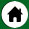# 初中数学知识点――二次函数解析式

1．二次函数y=ax&sup2;，y=a(x-h)&sup2;，y=a(x-h)&sup2;+k，y=ax&sup2;+bx+c(各式中，a≠0)的图象形状相同，只是位置不同，它们的顶点坐标及对称轴如下表：

解析式

y=ax&sup2;

y=a(x-h)&sup2;

y=a(x-h)&sup2;+k

y=ax&sup2;+bx+c

顶点坐标

&#91;0，0&#93;

&#91;h，0&#93;

&#91;h，k&#93;

&#91;-b/2a,(4ac-b&sup2;)/4a&#93;

对称轴

x=0

x=h

x=h

x=-b/2a

当h<0时，则向左平行移动|h|个单位得到．

当h>0,k>0时，将抛物线y=ax&sup2;向右平行移动h个单位，再向上移动k个单位，即可得

当h>0,k<0时，将抛物线y=ax&sup2;向右平行移动h个单位，再向下移动|k|个单位可得到y=a(x-h)&sup2;+k的图象；

当h<0,k>0时，将抛物线向左平行移动|h|个单位，再向上移动k个单位可得到y=a(x-h)&sup2;+k的图象；

当h<0,k<0时，将抛物线向左平行移动|h|个单位，再向下移动|k|个单位可得到y=a(x-h)&sup2;+k的图象；

因此，研究抛物线y=ax2+bx+c(a≠0)的图象，通过配方，将一般式化为y=a(x-h)&sup2;+k的形式，可确定其顶点坐标、对称轴，抛物线的大体位置就很清楚了．这给画图象提供了方便．

2．抛物线y=ax&sup2;+bx+c(a≠0)的图象：当a>0时，开口向上"当a<0时,开口向下，对称轴是直线x=-b/2a，顶点坐标是&#91;-b/2a,(4ac-b&sup2;)/4a&#93;

3．抛物线y=ax&sup2;+bx+c(a≠0)，若a>0，当x≤-b/2a时，y随x的增大而减小；当x≥-b/2a时，y随x的增大而增大．若a<0，当x≤-b/2a时，y随x的增大而增大；当x≥-b/2a时，y随x的增大而减小．4．抛物线y=ax&sup2;+bx+c的图象与坐标轴的交点：

(1)图象与y轴一定相交，交点坐标为(0，c)；

(2)当△=b2-4ac>0，图象与x轴交于两点A(x1,0)和B(x2,0)，其中的x1,x2是一元二次方程ax&sup2;+bx+c=0

(a≠0)的两根．这两点间的距离AB=|x2-x1|=．

当△=0．图象与x轴只有1个交点；

当△<0．图象与x轴没有交点．当a>0时，图象落在x轴的上方，x为任何实数时，都有y>0；当a<0时，图象落在x轴的下方，x为任何实数时，都有y<0．

5．抛物线y=ax&sup2;+bx+c的最值：如果a>0(a<0)，则当x=时，y最小(大)值=．

顶点的横坐标，是取得最值时的自变量值，顶点的纵坐标，是最值的取值．

6．用待定系数法求二次函数的解析式

(1)当题给条件为已知图象经过3个已知点或已知x、y的三对对应值时，可设解析式为一般形式：

y=ax&sup2;+bx+c(a≠0)．

(2)当题给条件为已知图象的顶点坐标或对称轴时，可设解析式为顶点式：y=a(x-h)&sup2;+k(a≠0)．

(3)当题给条件为已知图象与x轴的2个交点坐标时，可设解析式为两根式：y=a(x-x1)(x-x2)(a≠0)．

7．二次函数知识很容易与其它知识综合应用，而形成较为复杂的综合题目。因此，以二次函数知识为主的综合性题目是中考的热点考题，往往以大题形式出现．

顶点坐标_顶点坐标-二次函数常用的一般形式

1.y=ax^2+bx+c（a≠0）

2.y=ax^2（a≠0）

3.y=ax^2+c（a≠0）

4.y=a(x-h)^2（a≠0）

5.y=a(x-h)^2+k（a≠0）←顶点式

6.y=a(x-x1)(x-x2)（a≠0）←交点式